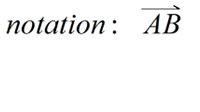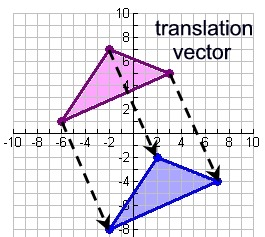# Translations and Vectors Worksheets

How Do Translations Relate to Vectors? A vector is typically represented by a directed line segment, the one with an arrowhead at one end indicating a direction. The directed line segment might look like a ray, but it has a specific length. The direction of the vector is represented from the initial point (tail) to the terminal point (head). fro example, if a vector starts from point A and terminates at point B, we can express the vector like:Also, we can write one letter to represent a vector. For instance, we can represent the same vector by using a letter u. The length of the vector is always expressed as the non-negative number. The direction of the vector is represented by the angle it makes with the horizontal line.The above figure shows the translation of the triangle on the coordinate plane. The translation of the triangle occurred at the 4 units to the right and 9 units down. The notation for this translation can be written as (4, -9). Vectors used for translating an object are known as the free vectors, which are the set of directed line segments. In translation, vectors that are parallel to each other and are of the same length are considered as identical. These vectors do not need to have the same starting and terminal points. In our example, the vectors connecting the image with the pre-image are of the same length and parallel to each other.

• ### Basic Lesson

Guides students through solving Translations & Vectors.

• ### Intermediate Lesson

Demonstrates the concept of advanced skill while solving Translations & Vectors.

• ### Independent Practice 1

A really great activity for allowing students to understand the concepts of the Translations & Vectors.

• ### Independent Practice 2

Students use Translations & Vectors in 20 assorted problems. The answers can be found below.

• ### Independent Practice 3

A really great activity for allowing students to understand the concepts of the Translations & Vectors.

• ### Independent Practice 4

Students use Translations & Vectors in 20 assorted problems. The answers can be found below.

• ### Homework

Students are provided with 12 problems to achieve the concepts of Translations & Vectors.

• ### Translations & Vectors Quiz

This tests the students ability to understand Translations & Vectors.